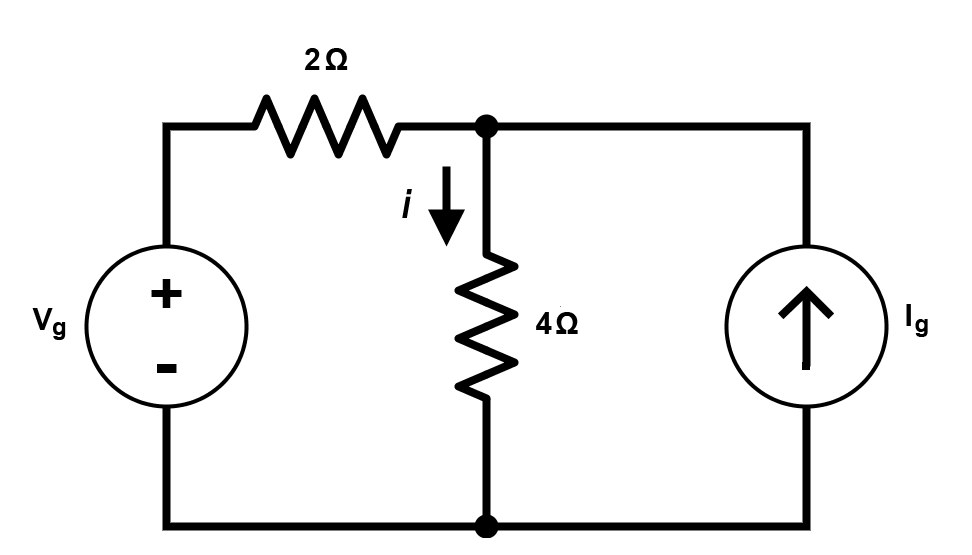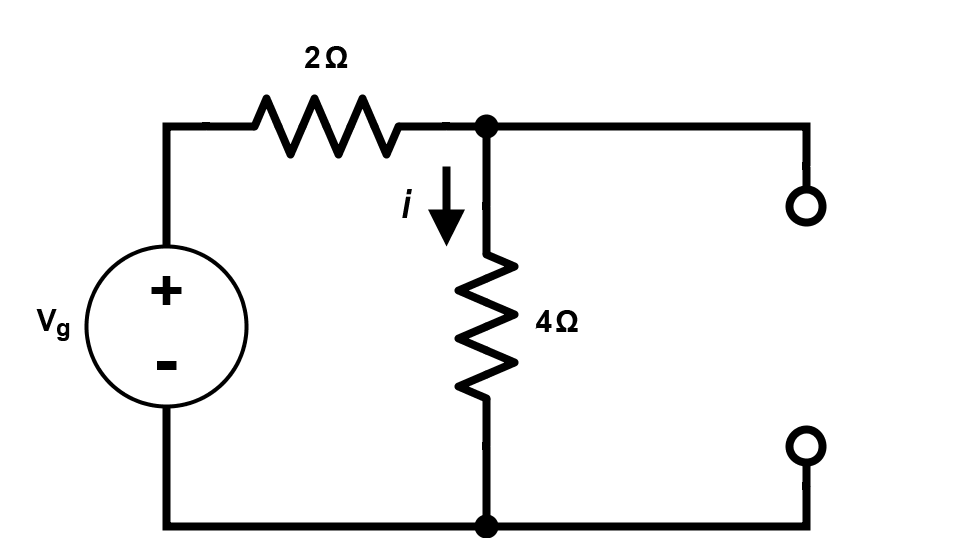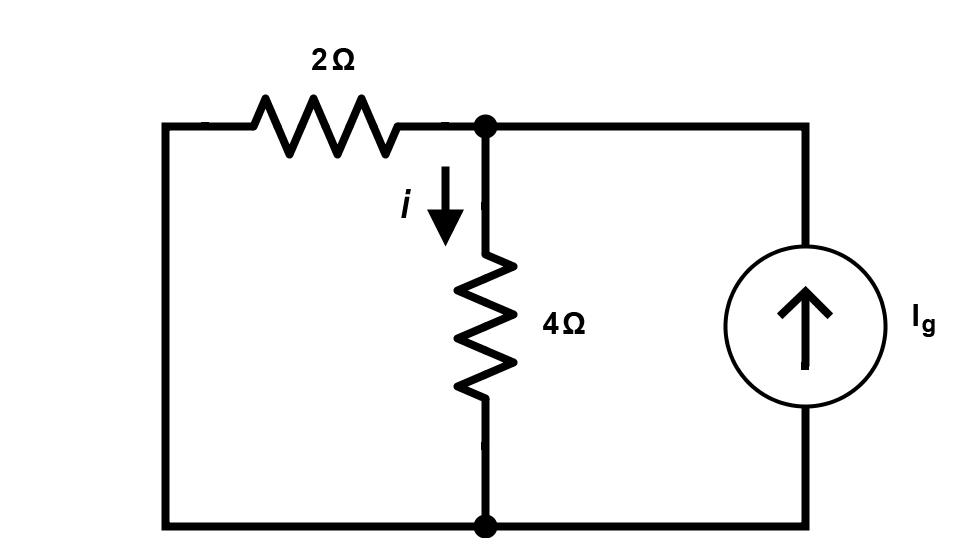Technical Article

# Superposition and What it Means For You

May 11, 2015 by Tristan Cornell

#### Recommended Level

Beginner

The concept of superposition, as it applies to electrical engineering, says that, in a linear circuit having more than one independent source, the total current in any part of the circuit equals the algebraic sum of the currents produced by each source separately. To calculate the current in a circuit with two or more sources, you have to analyze the circuit, taking each source separately. Basically you make separate circuits from the same schematic and interpret how each source affects the total current, then add the results. It sounds a lot more complicated than it is.

The figure below shows how you would analyze a circuit with a voltage source and a current source. To compute the contribution to i of the voltage source, you would treat the current source as being open, or an infinitely high output impedance. To compute the contribution from the current source, you'd treat the voltage source as a short circuit then compute what i would be. The total current would then be the sum of those two currents.$$2(i-I_{g})+4i=V_{g}$$

$$6i=V_{g}+2I_{g}$$

$$i=\frac{V_{g}}{6}+\frac{I_{g}}{3}$$

Note that $$\frac{V_{g}}{6}$$ is the component from the voltage source and $$\frac{I_{g}}{3}$$ is the component from the current source.$$i=\frac{V_{g}}{6}$$$$i=\frac{2I_{g}}{(2+4)}=\frac{2I_{g}}{6}=\frac{I_{g}}{3}$$

When doing this type of analysis, be careful that you consider the direction of the current flow from each source. If the current flows are in different directions, you'll have to subtract rather than add the currents.

#### Applications

The superposition principle can be applied to any electronic circuit with more than one independent source, even when one of the sources is an alternating current. By analyzing the AC and DC response separately, and then combining them using the principle of superposition, you can greatly simplify the analysis of complex circuits. For example, to analyze a transmitter with reactive components, such as inductors and capacitors, you need to calculate the circuit's response to AC sources whose output lies within a range of frequencies. When analyzing the response to DC sources, inductors will look like a short circuit and capacitors will look like an open circuit.